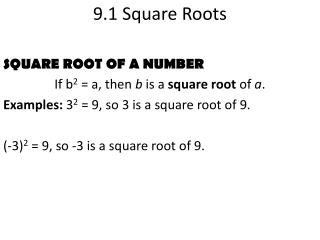DownloadDownload Presentation9.1 Square Roots

# 9.1 Square Roots

Download Presentation## 9.1 Square Roots

- - - - - - - - - - - - - - - - - - - - - - - - - - - E N D - - - - - - - - - - - - - - - - - - - - - - - - - - -
##### Presentation Transcript

1. 9.1 Square Roots SQUARE ROOT OF A NUMBER If b2 = a, then b is a square root of a. Examples:32= 9, so 3 is a square root of 9. (-3)2= 9, so -3 is a square root of 9.

2. Chapter 9 Test Review Evaluate the expression. -

3. Chapter 9 Test Review Evaluate the expression.

4. Chapter 9 Test Review Evaluate the expression.

5. Chapter 9 Test Review Evaluate the expression. -

6. 9.2 Solving Quadratic Equations by Finding Square Roots QUADRATIC EQUATION When b = 0, this equation becomes ax2 + c = 0. One way to solve a quadratic equation of the form ax2+ c = 0 is to isolate the x2 on one side of the equation. Then find the square root(s) of each side.

7. Chapter 9 Test Review Solve the equation. x2 = 144

8. Chapter 9 Test Review Solve the equation. 8x2= 968

9. Chapter 9 Test Review Solve the equation. 5x2 – 80 = 0

10. Chapter 9 Test Review Solve the equation. 3x2 – 4 = 8

11. 9.3 Simplifying Radicals PRODUCT PROPERTY OF RADICALS = EXAMPLE: = = = 2

12. Chapter 9 Test Review Simplify the expression.

13. Chapter 9 Test Review Simplify the expression.

14. Chapter 9 Test Review Simplify the expression.

15. Chapter 9 Test Review Simplify the expression.

16. 9.5 Solving Quadratic Equations by Graphing The x-intercepts of graphy = ax2 + bx + c are the solutions of the related equations ax2 + bx + c = 0. Recall that an x-intercept is the x-coordinate of a point where a graph crosses the x-axis. At this point, y = 0.

17. Chapter 9 Test Review Use a graph to estimate the solutions of the equation. Check your solutions algebraically. x2 – 3x = -2

18. Chapter 9 Test Review Use a graph to estimate the solutions of the equation. Check your solutions algebraically. -x2 + 6x = 5

19. Chapter 9 Test Review Use a graph to estimate the solutions of the equation. Check your solutions algebraically. x2 – 2x = 3

20. 9.6 Solving Quadratic Equations by the Quadratic Formula The solutions of the quadratic equation ax2 + bx + c = 0 are: x = when a ≠ 0 and b2 – 4ac > 0. THE QUADRATIC FORMULA

21. Chapter 9 Test Review Use the quadratic formula to solve the equation. 3x2 – 4x + 1 = 0

22. Chapter 9 Test Review Use the quadratic formula to solve the equation. -2x2 + x + 6 = 0

23. Chapter 9 Test Review Use the quadratic formula to solve the equation. 10x2– 11x + 3 = 0

24. 9.7 Using the Discriminant In the quadratic formula, the expression inside the radical is the DISCRIMINANT. x = DISCRIMINANT - 4ac

25. Chapter 9 Test Review Find the value of the discriminant. Then determine whether the equation has two solutions, one solution, or no real solution. 3x2 – 12x + 12 =0

26. Chapter 9 Test Review Find the value of the discriminant. Then determine whether the equation has two solutions, one solution, or no real solution. 2x2 + 10x + 6=0

27. Chapter 9 Test Review Find the value of the discriminant. Then determine whether the equation has two solutions, one solution, or no real solution. -x2 + 3x - 5 =0